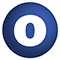# Sorting

#### object Sorting

The Sorting object provides functions that can sort various kinds of objects. You can provide a comparison function, or you can request a sort of items that are viewable as scala.math.Ordered. Some sorts that operate directly on a subset of value types are also provided. These implementations are derived from those in the Sun JDK.

Note that stability doesn't matter for value types, so use the `quickSort` variants for those. `stableSort` is intended to be used with objects when the prior ordering should be preserved, where possible.

Version

1.0

Linear Supertypes
AnyRef, Any
Ordering
1. Alphabetic
2. By inheritance
Inherited
1. Sorting
2. AnyRef
3. Any
1. Hide All
2. Show all
Visibility
1. Public
2. All

### Value Members

1. #### final def !=(arg0: Any): Boolean

Definition Classes
AnyRef → Any
2. #### final def ##(): Int

Definition Classes
AnyRef → Any
3. #### final def ==(arg0: Any): Boolean

Definition Classes
AnyRef → Any
4. #### final def asInstanceOf[T0]: T0

Definition Classes
Any
5. #### def clone(): AnyRef

Attributes
protected[java.lang]
Definition Classes
AnyRef
Annotations
( ... )
6. #### final def eq(arg0: AnyRef): Boolean

Definition Classes
AnyRef
7. #### def equals(arg0: Any): Boolean

Definition Classes
AnyRef → Any
8. #### def finalize(): Unit

Attributes
protected[java.lang]
Definition Classes
AnyRef
Annotations
( classOf[java.lang.Throwable] )
9. #### final def getClass(): Class[_]

Definition Classes
AnyRef → Any
10. #### def hashCode(): Int

Definition Classes
AnyRef → Any
11. #### final def isInstanceOf[T0]: Boolean

Definition Classes
Any
12. #### final def ne(arg0: AnyRef): Boolean

Definition Classes
AnyRef
13. #### final def notify(): Unit

Definition Classes
AnyRef
14. #### final def notifyAll(): Unit

Definition Classes
AnyRef
15. #### def quickSort(a: Array[Float]): Unit

Quickly sort an array of Floats.

16. #### def quickSort(a: Array[Int]): Unit

Quickly sort an array of Ints.

17. #### def quickSort[K](a: Array[K])(implicit arg0: math.Ordering[K]): Unit

Quickly sort an array of items with an implicit Ordering.

18. #### def quickSort(a: Array[Double]): Unit

Quickly sort an array of Doubles.

19. #### def stableSort[K, M](a: Seq[K], f: (K) ⇒ M)(implicit arg0: ClassTag[K], arg1: math.Ordering[M]): Array[K]

Stably sorts a sequence of items given an extraction function that will return an ordered key from an item.

Stably sorts a sequence of items given an extraction function that will return an ordered key from an item.

a

the sequence to be sorted.

f

the comparison function.

returns

the sorted sequence of items.

20. #### def stableSort[K](a: Seq[K])(implicit arg0: ClassTag[K], arg1: math.Ordering[K]): Array[K]

Sorts an arbitrary sequence of items that are viewable as ordered.

21. #### def stableSort[K](a: Seq[K], f: (K, K) ⇒ Boolean)(implicit arg0: ClassTag[K]): Array[K]

Sorts an arbitrary sequence into an array, given a comparison function that should return `true` iff parameter one is strictly less than parameter two.

Sorts an arbitrary sequence into an array, given a comparison function that should return `true` iff parameter one is strictly less than parameter two.

a

the sequence to be sorted.

f

the comparison function.

returns

the sorted sequence of items.

22. #### def stableSort[K](a: Array[K], f: (K, K) ⇒ Boolean)(implicit arg0: ClassTag[K]): Unit

Sorts an array of `K` given an ordering function `f`.

Sorts an array of `K` given an ordering function `f`. `f` should return `true` iff its first parameter is strictly less than its second parameter.

23. #### def stableSort[K](a: Array[K])(implicit arg0: ClassTag[K], arg1: math.Ordering[K]): Unit

Sort an array of K where K is Ordered, preserving the existing order where the values are equal.

24. #### final def synchronized[T0](arg0: ⇒ T0): T0

Definition Classes
AnyRef
25. #### def toString(): String

Definition Classes
AnyRef → Any
26. #### final def wait(): Unit

Definition Classes
AnyRef
Annotations
( ... )
27. #### final def wait(arg0: Long, arg1: Int): Unit

Definition Classes
AnyRef
Annotations
( ... )
28. #### final def wait(arg0: Long): Unit

Definition Classes
AnyRef
Annotations
( ... )